# JP5189451B2 - Projection lens and projection-type image display device - Google Patents

## Info

Publication number
JP5189451B2
JP5189451B2 JP2008254991A JP2008254991A JP5189451B2 JP 5189451 B2 JP5189451 B2 JP 5189451B2 JP 2008254991 A JP2008254991 A JP 2008254991A JP 2008254991 A JP2008254991 A JP 2008254991A JP 5189451 B2 JP5189451 B2 JP 5189451B2
Authority
JP
Japan
Prior art keywords
lens
projection
lenses
refractive power
projection lens
Prior art date
Legal status (The legal status is an assumption and is not a legal conclusion. Google has not performed a legal analysis and makes no representation as to the accuracy of the status listed.)
Expired - Fee Related
Application number
JP2008254991A
Other languages
Japanese (ja)
Other versions
JP2010085732A (en
Inventor

Original Assignee
リコー光学株式会社
Priority date (The priority date is an assumption and is not a legal conclusion. Google has not performed a legal analysis and makes no representation as to the accuracy of the date listed.)
Filing date
Publication date
Application filed by リコー光学株式会社 filed Critical リコー光学株式会社
Priority to JP2008254991A priority Critical patent/JP5189451B2/en
Publication of JP2010085732A publication Critical patent/JP2010085732A/en
Application granted granted Critical
Publication of JP5189451B2 publication Critical patent/JP5189451B2/en
Expired - Fee Related legal-status Critical Current
Anticipated expiration legal-status Critical

• 230000003287 optical Effects 0.000 claims description 17
• 230000005499 meniscus Effects 0.000 claims description 15
• 239000006185 dispersion Substances 0.000 claims description 6
• 230000004075 alteration Effects 0.000 description 58
• 238000010586 diagram Methods 0.000 description 16
• 201000009310 astigmatism Diseases 0.000 description 15
• 206010010071 Coma Diseases 0.000 description 12
• 230000014509 gene expression Effects 0.000 description 9
• 239000004973 liquid crystal related substance Substances 0.000 description 6
• 206010073261 Ovarian theca cell tumour Diseases 0.000 description 5
• 208000001644 Thecoma Diseases 0.000 description 5
• 239000006059 cover glass Substances 0.000 description 5
• 230000002194 synthesizing Effects 0.000 description 5
• 230000015572 biosynthetic process Effects 0.000 description 4
• 238000003786 synthesis reaction Methods 0.000 description 4
• 239000011521 glass Substances 0.000 description 3
• 239000003086 colorant Substances 0.000 description 2
• 239000002131 composite material Substances 0.000 description 1
• 239000000463 material Substances 0.000 description 1
• 239000000203 mixture Substances 0.000 description 1
• 239000005304 optical glass Substances 0.000 description 1
• 239000005342 prism glass Substances 0.000 description 1
• 230000001681 protective Effects 0.000 description 1
• 238000004904 shortening Methods 0.000 description 1
• 230000001629 suppression Effects 0.000 description 1

## Images

•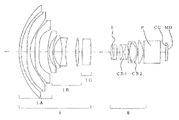•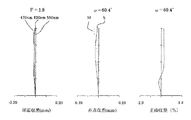•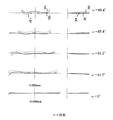•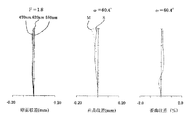•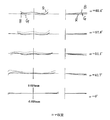•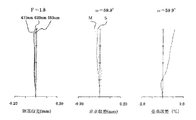•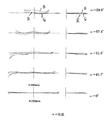••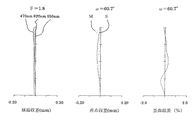•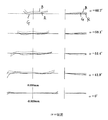•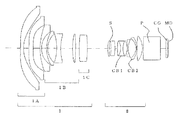•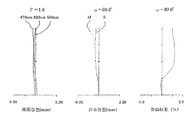•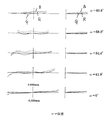•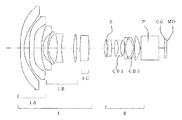•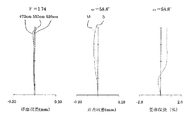•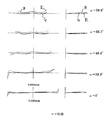•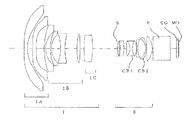•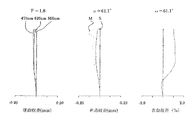•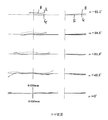## Description

The present invention relates to a projection lens and a projection type image display device equipped with the projection lens.

A projector that enlarges and projects a small original image displayed on a liquid crystal display device, DMD (digital mirror device), etc. onto a screen can easily obtain a large image, and in particular, images of three primary colors such as red, green, and blue. A “three-plate projector that individually displays components on three liquid crystal display elements” is widely used because a color image to be enlarged and projected is high definition.

In this type of projector, a “prism” must be placed between the projection lens and the liquid crystal display element as an optical system for synthesizing the three primary colors such as red, green, and blue emitted from the three liquid crystal display elements. In addition, a “long back focus” is required for the projection lens in order to secure a prism arrangement space.

Further, when the angle of light rays incident on the prism from the liquid crystal display element changes, the brightness of each color in the projected color image changes depending on the angle of view, resulting in an image that is difficult to see. In order to avoid this, it is preferable that the projection lens has a “telecentric” property in which the angle of the principal ray is substantially parallel to the optical axis on the reduction side.

As a projection lens having the optical function as described above, a so-called “retro focus type” lens in which a “negative refractive power lens group” and a “positive refractive power lens group” are arranged from the enlargement side has been conventionally used. As is known, distortion and lateral chromatic aberration are likely to occur significantly because the refractive power arrangement on the enlargement side and the reduction side is asymmetric.

Furthermore, recently, there has been a strong demand for “projection of the same large screen as before with a short projection distance”, and in order to respond to this, the focal length of the projection lens has to be “shorter”. The design of industrial lenses is becoming increasingly difficult.

There is known a device for reducing such a problem and realizing a retrofocus type projection lens having a wide angle of view (Patent Documents 1 and 2), but there is a demand for a large projection screen required for the projector. There is a demand for the realization of a projection lens that is becoming increasingly higher, has a high angle of view, and is bright (small F number).

Japanese Laid-Open Patent Publication No. 2007-147970 JP 2007-256712 A

In view of the circumstances as described above, the present invention realizes a projection lens having a wide angle of view, a sufficiently bright F number, a long back focus and high telecentricity, and capable of obtaining a good image with small aberrations. An object of the present invention is to realize a projection-type image display device that can project a high-definition image using a lens over a large area.

As illustrated in FIG. 1, the projection lens of the present invention has a “first lens group I having negative refractive power” from the enlargement side (left side in FIG. 1) with the largest air interval in the lens system as a boundary. And “second lens group II having a positive refractive power” are arranged, and is telecentric on the reduction side (the right side of the figure).

The first lens group I is formed by arranging the 1A group, 1B group, and 1C group indicated by reference numerals 1A, 1B, and 1C in the drawing in the above order from the magnification side.

In addition, in FIG.1, FIG.8, FIG.11, FIG.14, FIG. 17 which shows the lens structural example of the projection lens of this invention, the 1A group, 1B group, 1C group in the wording described in the claim, The three cemented lenses CB1 and CB2 are denoted by reference numerals 1A, 1B, 1C, CB1, and CB2.

The 1A group on the most enlarged side includes two “lenses having an aspheric surface”.
In the 1B group, "two meniscus lenses with a convex surface facing the enlargement side and negative refractive power", "lens with both sides concave", and "lens with both sides convex" are arranged in the above order from the enlargement side. It becomes.
The 1C group includes at least one “lens having a positive refractive power”.

The second lens group II includes “one lens having an aspheric surface and two three cemented lenses in which three lenses are bonded” from the enlargement side to the reduction side.
Then, “back focus in air when the conjugate point on the enlargement side is at infinity”: Bf, focal length of the entire system: f, focal length of the first lens group I: f1, focal length of the second lens group II : F2 is the condition:
(1) 3.0 <Bf / f <5.0
(2) 8.0 <| f1 / f | <20.0
(3) 4.5 <f2 / f <6.5
(Claim 1).

In FIG. 1, symbol S indicates “aperture stop”, symbol P indicates “prism as a color synthesis optical system”, symbol CG indicates “cover glass synthesis system”, and symbol MD indicates “display of liquid crystal display elements and the like”. Element ".

The projection lens according to claim 1 is a composite focal length of “three lenses having negative refractive power” continuously arranged in the 1B group in the first lens group I: f1Bn, the first lens group. I focal length: f1 is the condition:
(4) 0.14 <f1Bn / f1 <0.35
Is preferably satisfied (claim 2).

3. The projection lens according to claim 1, wherein the two three-piece cemented lenses in the second lens group include three-piece cemented lenses CB <b> 1 having a positive refractive power on the enlargement side, as illustrated in FIG. 1. The reduction side can be a three-piece cemented lens CB2 having negative refractive power.

Of the three-piece cemented lenses CB1 and CB2 arranged in this way, the three-piece cemented lens CB1 has a “lens L1 having a positive refractive power” and a “lens L2 having both concave surfaces” from the enlargement side toward the reduction side. ”,“ Lens with convex on both sides L3 ”, and the three-piece cemented lens CB2 has“ Lens L4 with negative refractive power ”,“ Lens L5 with convex on both sides ”,“ Meniscus ” The lens L6 "having a negative refracting power in shape can be composed of three lenses (claim 4).

In the projection lens according to claim 4, the refractive index of the lens L2 included in the three-piece cemented lens CB1: N L2 , Abbe number: ν L2 , and the refractive index of the lens L3 with respect to d line: N L3 , Abbe number. : Ν L3 , but conditions:
(5) 0.3 <N L2 −N L3 <0.5
(6) 40 <ν L3 −ν L2 <60
Is preferably satisfied (Claim 5).

In the projection lens according to claim 4, the Abbe number: ν L1 and the partial dispersion ratio: θ gF of the lens L1 included in the three-piece cemented lens CB1 are as follows:
(7) 0 <θ gF − (0.6438−0.001682ν L1 ) <0.05
Is preferably satisfied (claim 6).

The projection lens according to any one of claims 4 to 6, wherein each of the refractive indexes: N L4 , N L5 , N L6 , with respect to the d-line of the lenses L4, L5, L6 included in the three-piece cemented lens CB2, Abbe number: ν L4 , ν L5 , ν L6
(8) 0.3 <(N L4 + N L6 ) / 2−N L5 <0.5
(9) 35 <ν L5 − (ν L4 + ν L6 ) / 2 <60
Is preferably satisfied (claim 7).

The projection lens according to any one of claims 1 to 7 may be focused by moving the 1B group and the 1C group in the first lens group I on the optical axis (claim 8). In this case, it is preferable that the conjugate point on the enlargement side is “moved from a long distance to a short distance to perform focusing” by expanding the distance between the 1B group and the 1C group and moving both to the reduction side. .

The projection lens according to any one of claims 1 to 9, wherein a lens having an aspheric surface in the second lens group is made of plastic, and its focal length: f P is a condition:
(10) 10.0 <| f P / f2 |
Is preferably satisfied (claim 10).

Any projection lens according to one of claims 1 to 10, half angle (in degrees): omega, F number representing the brightness of the lens: F NO is the condition:
(11) 50 ° <ω <65 °
(12) 1.7 < FNO <2.5
Is preferably satisfied (claim 11).

A projection type image display device according to the present invention includes the projection lens according to any one of claims 1 to 11 (claim 12). Of course, the projection type image display apparatus can be implemented as a “three-plate projector”.
In order to obtain a projection lens that can project a large screen with a short projection distance, it is necessary to make the focal length shorter than that of a conventional projection lens. The focal length of the entire projection lens system decreases as the “lens surface interval / radius of curvature” is reduced as it is, but shortening the focal length by this method increases the focal length as it shortens. Aberration occurs, image quality is deteriorated, and back focus is shortened.

As illustrated in FIG. 1, the projection lens of the present invention has a first lens group I having “negative refractive power” on the enlargement side and “positive” on the reduction side, with the largest air interval in the lens system as a boundary. The second lens group II having “refractive power” is a retrofocus type.
The first lens group I includes a 1A group including two “lens having an aspherical surface”, “two meniscus lenses having a convex surface on the enlargement side and having negative refractive power, and lenses having concave both surfaces” , A lens having convex surfaces on both sides in the above order from the magnifying side, and a 1C group including at least one lens having a positive refractive power. The second lens group II has “aspherical surface 1 By adopting a configuration including a single lens and two three-lens cemented lenses, it is possible to effectively correct the aberration.

Condition (1) is a condition for securing a sufficient back focus necessary for the projection lens while maintaining a desired “large field angle”.
When the parameter: Bf / f exceeds the lower limit of the conditional expression (1) while maintaining a wide angle of view, the focal length of the entire system becomes long and the back focus: Bf becomes short, and the projection lens and the display element It becomes difficult to arrange a color synthesis optical system such as a prism between them.
If the upper limit of the condition (1) is exceeded while the desired “sufficient back focus” is maintained, the focal length f of the entire system becomes small, making it difficult to correct various aberrations. .

The condition (2) is a condition for “both sufficiently long back focus and good optical performance”.

If the parameter: | f1 / f | exceeds the upper limit of the condition (2), | f1 | becomes too large and the “negative refractive power” of the first lens group becomes small, and it is difficult to obtain a desired back focus. become.

If the lower limit of the condition (2) is exceeded, sufficient back focus can be secured, but | f1 | becomes too small and the “negative refractive power” of the first lens group becomes excessive, and coma aberration, curvature of field, etc. It is difficult to keep the aberration of the lens in good condition.

Condition (3) is a condition for “making both lens cost and good optical performance compatible”.
When the parameter: f2 / f exceeds the upper limit of the condition (3), the positive focal length: f2 of the second lens group increases and “back focus extends”, but the lens also increases accordingly, resulting in an increase in cost.
If the lower limit of condition (3) is exceeded, the focal length f2 of the second lens group becomes short, and the positive refractive power of the second lens group becomes excessive, making it difficult to correct various aberrations.

Condition (4) defines “distribution of negative refractive power to glass lens” in the first lens group, and is a condition for realizing good and stable optical performance.

In a specific example which will be described later, the large “two lenses having an aspheric surface” on the enlargement side of the 1A group arranged on the enlargement side in the first lens group are all made of plastic. Costs are kept down, but plastic is susceptible to temperature and humidity.

Parameter: By keeping f1Bn / f1 within the range of the condition (4), it is possible to avoid giving these plastic lenses a large refractive power, and to keep the refractive index fluctuations associated with changes in temperature and humidity to a small level. It is possible to stabilize and obtain a desired “large field angle” and “sufficient back focus”.

The two three-piece cemented lenses in the second lens group can have CB1 having a positive refractive power and CB2 having a negative refractive power from the enlargement side, as in claim 3. The cemented lens CB1 is composed of “three lenses of a lens L1 having a positive refractive power, a lens L2 having a concave surface on both sides, and a lens L3 having a convex surface on both sides”, and the three-unit cemented lens CB2 on the reduction side has a “negative refraction”. It is preferable that the lens is composed of a lens L4 having power, a lens L5 having convex surfaces on both sides, and a lens L6 having a meniscus shape and negative refractive power.

Conditions (5) and (6) define the refractive indices and Abbe numbers of the two lenses L2 and L3 in the three-piece cemented lens CB1, and conditional expressions (8) and (9) are three-piece cemented lenses: It defines the refractive index and Abbe number of the three lenses L4, L5, L6 in CB2.

When these parameters satisfy the conditions (5), (6), (8), and (9), it is possible to realize a projection lens having “good image performance with little lateral chromatic aberration and axial chromatic aberration”. .

In the projection lens according to the fifth aspect, the Abbe number and partial dispersion ratio of the lens: L1 arranged on the “most enlarged side” in the three-piece cemented lens: CB1 are defined by the conditional expression (6).

“Partial dispersion ratio: θ gF ” of optical glass is
Refractive index for g-line (435.83 nm): Ng,
Refractive index for F-line (486.13 nm): NF,
Refractive index for C-line (656.27 nm): NC,
By
θ gF = (Ng−NF) / (NF−NC)
It is represented by

Lens in the three-piece cemented lens CB1: Since the “Abbe number and partial dispersion ratio” of L1 satisfies the condition (7), the chromatic aberration of magnification of the projection lens can be further reduced, and a good image can be obtained over a wide angle of view. Can be obtained.

Focusing is generally performed by “moving the most magnified lens group to adjust the focus”. However, in the projection lens according to the present invention, the focusing lens is arranged inside the first lens group. The 1B group and the 1C group can be moved to focus. In this way, since it is not necessary to move the “large 1A group” arranged on the enlargement side, focusing can be performed with less driving energy, which is convenient.

The projection lens according to claim 9 has a narrow projection distance range in which a good image can be obtained because the higher the angle of view, the more easily the balance between the curvature of the image and the distortion is distorted by the change in the projection distance. The lens for the image has good curvature and distortion of the image plane by moving the projectors projecting on a distant screen closer to the screen by “moving them together while expanding the distance between the 1B group and the 1C group” Focusing is possible while keeping

A lens disposed in the second lens group and having an aspherical surface (in the projection lens illustrated in FIG. 1, the tenth lens from the magnification side, that is, the lens closest to the magnification side of the three-piece cemented lens CB1) is plastic. However, the plastic has a large “change in refractive index due to temperature”, and the position of the focus is likely to change with changes in temperature.
The condition (10) is a condition for restricting the focal length of the aspherical lens formed of plastic and “suppressing the fluctuation of the focus position due to the temperature change”.

When the parameter: | f P / f2 | becomes smaller than the lower limit of the condition (10), the plastic lens becomes a “lens with a short focal length and a strong power”, and the projection lens has a “change in focus position due to a temperature change”. It will be “big”.

By satisfying the condition (11), it is possible to “realize a projection image of a desired size at a short projection distance with a large half angle of view”. In addition, when the “projection image has the same size as the conventional one”, the display element can be changed to “smaller”, so that “the display element can move within the image circle”. The image can be shifted and projected in a “direction that does not get in the way”.

When the condition (12) is satisfied, a sufficiently bright projection image can be provided to the observer.

As described above, according to the present invention, it is possible to provide a novel projection lens and a projection-type image display device equipped with the projection lens.
The projection lens of the present invention has a back focus close to 30 mm with a short focal length of around 7 mm, a half angle of view exceeding 60 degrees, and an F number of about 1.8, as shown in the examples described later. It is possible to achieve bright and good performance.

An embodiment will be described.
The projection lens whose embodiment is shown in FIG. 1 relates to Example 1 described later.

The projection lens of this embodiment has a first lens group I having a negative refractive power and a second lens group II having a positive refractive power from the enlargement side with the largest air interval in the lens system as a boundary. Are arranged in the above order and are telecentric on the reduction side.
The first lens group I includes, in order from the magnifying side, a 1A group including two lenses having aspherical surfaces, “a meniscus shape having a convex surface on the magnifying side and two lenses having negative refractive power and concave on both surfaces. 1B group in which “a lens and a convex lens on both sides” are arranged, and 1C group including at least one lens having positive refractive power.

In this embodiment, the 1A group includes three lenses, and the 1C group includes one biconvex lens.
The second lens group includes one lens having an aspherical surface and three cemented lenses CB1 and CB2 in which the three lenses are bonded together. A “positive lens on the enlargement side and a negative lens on the reduction side” are arranged on the enlargement side of the three-piece cemented lens CB1, and one positive lens is arranged on the reduction side of the three-piece cemented lens CB2.
Example 1 that embodies this embodiment satisfies the conditions (1) to (12).

The projection lens whose embodiment is shown in FIG. 8 relates to Example 2 described later.

The projection lens of this embodiment has a first lens group I having a negative refractive power and a second lens group II having a positive refractive power from the enlargement side with the largest air interval in the lens system as a boundary. Are arranged in the above order and are telecentric on the reduction side.
The first lens group I includes, in order from the magnifying side, a 1A group including two lenses having aspherical surfaces, “a meniscus shape having a convex surface on the magnifying side and two lenses having negative refractive power and concave on both surfaces. 1B group in which “a lens and a convex lens on both sides” are arranged, and 1C group including at least one lens having positive refractive power.

The second lens group includes one lens having an aspherical surface and three cemented lenses CB1 and CB2 in which the three lenses are bonded together. A “positive lens on the enlargement side and a negative lens on the reduction side” are arranged on the enlargement side of the three-piece cemented lens CB1, and one positive lens is arranged on the reduction side of the three-piece cemented lens CB2.
Example 2 which embodies this embodiment satisfies the conditions (1) to (12).

The projection lens whose embodiment is shown in FIG. 11 relates to Example 3 to be described later.

The projection lens of this embodiment has a first lens group I having a negative refractive power and a second lens group II having a positive refractive power from the enlargement side with the largest air interval in the lens system as a boundary. Are arranged in the above order and are telecentric on the reduction side.
The first lens group I includes, in order from the magnifying side, a 1A group including two lenses having aspherical surfaces, “a meniscus shape having a convex surface on the magnifying side and two lenses having negative refractive power and concave on both surfaces. 1B group in which “a lens and a convex lens on both sides” are arranged, and 1C group including at least one lens having positive refractive power.

The second lens group includes one lens having an aspherical surface and three cemented lenses CB1 and CB2 in which the three lenses are bonded together. A “positive lens on the enlargement side and a negative lens on the reduction side” are arranged on the enlargement side of the three-piece cemented lens CB1, and one positive lens is arranged on the reduction side of the three-piece cemented lens CB2.
Example 3 which embodies this embodiment satisfies the conditions (1) to (12).

The projection lens whose embodiment is shown in FIG. 14 relates to Example 4 to be described later.

The projection lens of this embodiment has a first lens group I having a negative refractive power and a second lens group II having a positive refractive power from the enlargement side with the largest air interval in the lens system as a boundary. Are arranged in the above order and are telecentric on the reduction side.
The first lens group I includes, in order from the magnifying side, a 1A group including two lenses having aspherical surfaces, “a meniscus shape having a convex surface on the magnifying side and two lenses having negative refractive power and concave on both surfaces. 1B group in which “a lens and a convex lens on both sides” are arranged, and 1C group including at least one lens having positive refractive power.

The second lens group includes one lens having an aspherical surface and three cemented lenses CB1 and CB2 in which the three lenses are bonded together. A “positive lens on the enlargement side and a negative lens on the reduction side” are arranged on the enlargement side of the three-piece cemented lens CB1, and one positive lens is arranged on the reduction side of the three-piece cemented lens CB2.
Example 4 which materializes this embodiment satisfies the conditions (1) to (12).

The projection lens whose embodiment is shown in FIG. 17 relates to Example 5 described later.

The projection lens of this embodiment has a first lens group I having a negative refractive power and a second lens group II having a positive refractive power from the enlargement side with the largest air interval in the lens system as a boundary. Are arranged in the above order and are telecentric on the reduction side.
The first lens group I includes, in order from the magnifying side, a 1A group including two lenses having aspherical surfaces, “a meniscus shape having a convex surface on the magnifying side and two lenses having negative refractive power and concave on both surfaces. 1B group in which “a lens and a convex lens on both sides” are arranged, and 1C group including at least one lens having positive refractive power.

The second lens group includes one lens having an aspherical surface and three cemented lenses CB1 and CB2 in which the three lenses are bonded together. A “positive lens on the enlargement side and a negative lens on the reduction side” are arranged on the enlargement side of the three-piece cemented lens CB1, and one positive lens is arranged on the reduction side of the three-piece cemented lens CB2.
Example 5 which embodies this embodiment satisfies the conditions (1) to (12).

The embodiment shown in FIGS. 8, 11, 14 and 17 differs from that of FIG. 1 in that the 1A group of the first lens group I is composed of two lenses having an aspheric surface. Is a configuration similar to that of FIG. However, in the embodiment of FIG. 17, the aperture stop S is placed in the vicinity of the enlargement side of the second lens group II.

In any of the embodiments shown in FIGS. 1, 8, 11, 14, and 17, the three-piece cemented lens CB1 in the second lens group is a lens L1 having a positive refractive power from the enlargement side. The lens is composed of three lenses, a lens L2 having a concave surface on both sides and a lens L3 having a convex surface on both sides. The three-piece cemented lens CB2 is a lens L4 having a negative refractive power, a lens L5 having a convex surface on both sides, and a meniscus negative shape. It consists of three lenses, a lens L6 having refractive power.

Hereinafter, five specific examples of the projection lens will be described.
In each example, “surface number” is represented by a number counted from the enlargement side (screen side) to the reduction side (display element side), and the screen is “object surface” and the display element is “image surface”.

“R” represents the radius of curvature of each surface (including the surface of the aperture stop S, the surface of the prism P for color synthesis, and the surface of the cover glass CG) (or the paraxial radius of curvature for an aspherical surface). "Represents the surface interval on the optical axis.

“Nd” and “νd” indicate the refractive index and Abbe number of the material of each lens with respect to the d-line.

“Effective diameter” indicates the maximum height of a light beam passing from the optical axis of the lens.

“Image height” is the maximum height of the display element surface from the optical axis, and “BF” is from the lens surface on the most reduced side in the air (without the prism and cover glass) when the conjugate point on the enlargement side is infinity. The distance to the paraxial image (back focus) is represented, and the “lens total length” is represented by adding the back focus to the distance from the most magnified lens surface to the most demagnified lens surface.

The shape of the aspherical surface is that the intersection with the optical axis is the origin, the height from the optical axis: h, the change in the optical axis direction: Z, the paraxial radius of curvature: R, the conic constant: K, the aspherical coefficient of the nth order term : An well known formula:
Z = (1 / R) · h 2 / [1 + √ {1- (1 + K) · (1 / R) 2 · h 2 }]
+ A4 ・ h 4 + A6 ・ h 6 + A8 ・ h 8 + ・ ・ ・ + An ・ h n
The shape is specified by giving the above R, K, An.

An aspheric surface is indicated by adding “*” to the surface number. Also. Throughout the examples, the unit of length dimension is “mm”.

FIG. 1 shows the lens configuration of the projection lens of Example 1.

The projection lens of Example 1 includes a first lens group I and a second lens group II from the magnification side (left side in the figure), and the aperture stop S is the first from the magnification side in the second lens group. It is placed between the second lens.

A prism P, which is a color composition system, and a cover glass CG are inserted between the projection lens and the display element MD. The 1A group arranged on the most magnified side includes a glass lens having a weak negative refractive power and two plastic aspherical lenses.

The 1B group has two “meniscus negative lenses having a convex surface facing the enlargement side”, a concave lens on both sides, and a convex lens on both sides in order from the enlargement side. It consists of a positive lens.

The second lens group II is a lens in which the second lens from the magnifying side has an aspheric surface, and is followed by two three-piece cemented lenses CB1 and CB2.

Focusing is performed by moving the 1B group and the 1C group, but when approaching the screen, the interval between the 1B group and the 1C group increases, and both move to the reduction side.

The position of the moving group in the three forms with a projection distance of 1207.1 mm, 980 mm, and 525.1 mm is shown below as variable data.

Various data are representatively represented as 980 mm, which is an intermediate projection distance of the three forms.

"Example 1"
Surface number RD Nd νd Effective diameter object surface ∞ (980.000)
1 90.033 3.000 1.51680 64.2 65.590
2 75.018 8.699 59.599
3 * −2765.451 4.569 1.53159 55.7 55.986
4 * 43.223 7.401 49.173
5 * −1895.270 5.982 1.53159 55.7 44.000
6 * 2260.790 (variable) 32.433
7 86.143 2.000 1.83400 37.3 29.147
8 29.201 5.438 22.527
9 50.720 1.600 1.80610 33.3 22.480
10 26.410 12.363 19.559
11 −64.119 5.128 1.48749 70.4 19.373
12 74.840 15.540 18.725
13 93.856 4.500 1.72342 38.0 19.239
14 −180.437 (variable) 19.138
15 211.740 13.000 1.60342 38.0 17.764
16 −153.465 (variable) 16.062
17 39.086 2.535 1.84666 23.8 10.187
18 154.937 0.320 9.891
19 (Aperture) ∞ 2.674 9.890
20 * −32.969 4.505 1.53159 55.7 9.739
21 * −36.282 4.114 9.767
22 175.009 4.866 1.80809 22.8 9.775
23 −18.035 2.524 1.90366 31.3 9.733
24 24.381 5.405 1.49700 81.6 9.803
25 −27.263 0.300 10.000
26 −155.438 1.200 1.84666 23.8 10.528
27 28.068 11.068 1.49700 81.6 11.246
28 −16.697 1.391 1.83400 37.3 12.409
29 −27.450 0.737 13.904
30 197.880 7.256 1.61800 63.4 15.921
31 −27.534 1.500 16.186
32 ∞ 25.000 1.51680 64.2 15.359
33 ∞ 8.300 13.349
34 ∞ 2.500 1.51680 64.2 12.333
35 ∞ 1.000 12.132
Image plane ∞.
Projection distance 1207.600 980.000 525.100
D6 12.922 13.127 14.193
D14 5.047 5.120 5.532
D16 29.917 29.638 28.161.

"Aspherical data"
Third surface K = 0, A4 = 0.657529 × 10 −5 , A6 = −0.384140 × 10 −8 ,
A8 = 0.122557 × 10 −11 , A10 = −0.714075 × 10 −16 ,
A12 = −0.542252 × 10 −19 , A14 = 0.130841 × 10 −22
A16 = −0.774765 × 10 −27 , A18 = 0.158013 × 10 −31
A20 = −0.554708 × 10 −36
Fourth surface K = −0.467607, A4 = −0.965808 × 10 −6 , A6 = −0.424709 × 10 −9 ,
A8 = −0.857036 × 10 −13 , A10 = −0.758785 × 10 −17 ,
A12 = 0.138984 × 10 −19 , A14 = 0.200640 × 10 −23
A16 = −0.141838 × 10 −26 , A18 = −0.722752 × 10 −31
A20 = 0.233806 × 10 −34
5th surface K = 200, A4 = 0.563778 × 10 −5 , A6 = 0.410174 × 10 −9 ,
A8 = −0.226994 × 10 −12 , A10 = −0.151297 × 10 −15 ,
A12 = −0.132677 × 10 −19 , A14 = −0.618192 × 10 −23
A16 = 0.968703 × 10 −26 , A18 = −0.635751 × 10 −31
A20 = 0.459849 × 10 −34
6th surface K = 155, A4 = 0.136642 × 10 −4 , A6 = 0.803123 × 10 −9 ,
A8 = −0.966227 × 10 −11 , A10 = 0.164954 × 10 −13 ,
A12 = −0.845526 × 10 −17 , A14 = 0.292742 × 10 −21
A16 = 0.07069 × 10 −23 , A18 = 0.939371 × 10 −29
A20 = 0.109378 × 10 −30
20th surface K = 2.782085, A4 = 0.600078 × 10 −4 , A6 = 0.1000085 × 10 −6 ,
A8 = −0.176704 × 10 −8 , A10 = 0.136181 × 10 −10 ,
A12 = −0.428831 × 10 −13
21st surface K = 3.20199, A4 = 0.703742 × 10 −4 , A6 = 0.154441 × 10 −6 ,
A8 = −0.263786 × 10 −8 , A10 = 0.212726 × 10 −10 ,
A12 = −0.713271 × 10 −13 .

"Various data"
Focal length 6.688
F number 1.80
Half angle of view 60.4 °
Image height 12.000
BF 28.902
Total lens length 214.902.

"Parameter values for conditional expressions"
(1) Bf / f = 4.322
(2) | f1 / f | = 9.129
(3) f2 / f = 5.335
(4) f1Bn / f1 = 0.287
(5) N L2 −N L3 = 0.40666
(6) ν L3 −ν L2 = 50.3
(7) θ gF − (0.6438−0.001682ν L1 ) = 0.026
(8) (N L4 + N L6 ) / 2−N L5 = 0.34333
(9) ν L5 − (ν L4 + ν L6 ) /2=51.1
(10) | f P /f2|=36.050
“Ω” indicates the half angle of view of the condition (11), and “F NO ” indicates the F number of the condition (12). The same applies to the following embodiments.

FIG. 2 shows a diagram of spherical aberration, astigmatism, and distortion aberration, and FIG. 3 shows a coma aberration when the projection lens of Example 1 is set to an object distance of 980 mm and the reduction side is evaluated.

Each aberration diagram shows the aberration of green light having a wavelength of 550 nm, but the spherical aberration diagram and coma aberration diagram also show aberrations at wavelengths of 620 nm and 470 nm, representing red and blue light.
In the graph of coma aberration, light having a wavelength of 620 nm is represented by “R”, light having a wavelength of 550 nm is represented by “G”, and light having a wavelength of 470 nm is represented by “B”. The same applies to the coma aberration diagrams of the other examples.
Wavelength: 620 In the astigmatism diagram, S represents the sagittal image plane, and M represents the meridional image plane aberration.

FIG. 4 shows spherical aberration, astigmatism and distortion when the object distance is 1207.1 mm, FIG. 5 shows spherical aberration, astigmatism and distortion when the object distance is 525.1 mm. The distortion aberration is shown in FIG. 6, and the coma aberration is shown in FIG.

FIG. 8 shows the lens configuration of the projection lens of Example 2.

The 1A group arranged on the most enlarged side is composed of only two plastic aspherical lenses. Plastic is soft and easily scratched, but scratches can be prevented by attaching a transparent protective member such as glass to the front surface of the lens.

Focusing is performed by moving the 1B group and the 1C group as in the first embodiment.

"Example 2"
Surface number RD Nd νd Effective diameter object surface ∞ (980.000)
1 * −620.831 4.550 1.53159 55.7 56.878
2 * 42.998 7.610 47.856
3 * −1908.289 6.174 1.53159 55.7 42.868
4 * 43647.052 (variable) 31.977
5 85.377 2.000 1.83400 37.3 30.260
6 27.196 7.149 22.456
7 46.191 1.600 1.80610 33.3 21.930
8 27.126 13.186 19.421
9 −61.118 5.670 1.48749 70.4 18.777
10 71.320 17.156 18.075
11 91.982 4.342 1.72342 38.0 18.634
12 −202.537 (variable) 18.527
13 169.302 9.908 1.60342 38.0 17.335
14 −163.759 (variable) 16.062
15 36.209 2.395 1.84666 23.8 10.030
16 89.958 0.537 9.711
17 (Aperture) ∞ 1.121 9.708
18 * −36.279 5.301 1.53159 55.7 9.693
19 * −45.060 3.167 9.654
20 104.640 5.052 1.80809 22.8 9.718
21 −16.729 4.800 1.90366 31.3 9.679
22 24.170 5.600 1.49700 81.6 9.790
23 −25.646 0.300 10.000
24 −154.276 1.200 1.84666 23.8 10.543
25 26.842 11.726 1.49700 81.6 11.264
26 −16.750 1.529 1.83400 37.3 12.571
27 −28.298 0.300 14.202
28 183.273 7.658 1.61800 63.4 16.155
29 −27.576 1.500 16.454
30 ∞ 25.000 1.51680 64.2 15.571
31 ∞ 9.000 13.487
32 ∞ 2.500 1.51680 64.2 12.344
33 ∞ 1.000 12.135
Image plane ∞.
Projection distance 1207.900 980.000 531.00
D4 9.906 9.980 11.045
D12 4.544 4.657 5.000
D14 29.800 29.613 28.205.

"Aspherical data"
First surface K = 0, A4 = 0.657242 × 10 −5 , A6 = −0.385244 × 10 −8 ,
A8 = 0.122384 × 10 −11 , A10 = −0.721103 × 10 −16 ,
A12 = −0.545054 × 10 −19 , A14 = 0.13032 × 10 −22
A16 = −0.789402 × 10 −27 , A18 = 0.192909 × 10 −31
A20 = 0.818963 × 10 −36
Second surface K = −0.457487, A4 = −0.11014 × 10 −5 , A6 = −0.434059 × 10 −9 ,
A8 = −0.759156 × 10 −13 , A10 = −0.527898 × 10 −18 ,
A12 = 0.138377 × 10 −19 , A14 = 0.196923 × 10 −23
A16 = −0.147645 × 10 −26 , A18 = −0.125703 × 10 −30
A20 = 0.1112002 × 10 −34
Third surface K = 200, A4 = 0.575386 × 10 −5 , A6 = 0.454239 × 10 −9 ,
A8 = −0.245963 × 10 −12 , A10 = −0.17235 × 10 −15 ,
A12 = −0.128878 × 10 −19 , A14 = −0.585419 × 10 −23
A16 = 0.979046 × 10 −26 , A18 = 0.545743 × 10 −30
A20 = −0.529688 × 10 −34
Fourth surface K = 155, A4 = 0.136642 × 10 −4 , A6 = 0.803123 × 10 −9 ,
A8 = −0.966227 × 10 −11 , A10 = 0.165596 × 10 −13 ,
A12 = −0.851609 × 10 −17 , A14 = 0.237916 × 10 −21
A16 = 0.998834 × 10 −24 , A18 = −0.832881 × 10 −28
A20 = −0.110126 × 10 −31
18th surface K = 3.239863, A4 = 0.555543 × 10 −4 , A6 = 0.900284 × 10 −7 ,
A8 = −0.196904 × 10 −8 , A10 = 0.156508 × 10 −10 ,
A12 = −0.496747 × 10 −13
19th surface K = 3.855361, A4 = 0.629564 × 10 −4 , A6 = 0.127848 × 10 −6 ,
A8 = −0.25756 × 10 −8 , A10 = 0.204455 × 10 −10 ,
A12 = −0.693496 × 10 −13 .

"Various data"
Focal length 6.630
F number 1.80
Half angle of view 60.7 °
Image height 12.000
BF 29.594
Total lens length 203.875.

"Parameter values for conditional expressions"
(1) Bf / f = 4.464
(2) | f1 / f | = 12.424
(3) f2 / f = 5.304
(4) f1Bn / f1 = 0.205
(5) N L2 −N L3 = 0.40666
(6) ν L3 −ν L2 = 50.3
(7) θ gF − (0.6438−0.001682ν L1 ) = 0.026
(8) (N L4 + N L6 ) / 2−N L5 = 0.34333
(9) ν L5 − (ν L4 + ν L6 ) /2=51.1
(10) | f P / f 2 | = 12.600.

FIG. 9 shows spherical aberration, astigmatism and distortion when the projection lens of Example 2 is set at an object distance of 980 mm and the reduction side is evaluated. FIG. 10 shows coma aberration.

FIG. 11 shows the lens configuration of the projection lens of Example 3.

"Example 3"
Surface number RD Nd νd Effective diameter object surface ∞ (980.000)
1 * −988.142 4.560 1.53159 55.7 57.308
2 * 43.026 7.732 48.808
3 * −1969.613 6.164 1.53159 55.7 43.049
4 * 21419.959 (variable) 32.226
5 87.270 2.000 1.83400 37.3 30.957
6 27.915 7.389 22.980
7 48.199 1.704 1.80610 33.3 22.420
8 27.143 13.074 19.671
9 −62.246 5.770 1.48749 70.4 19.171
10 70.787 16.977 18.401
11 89.819 4.500 1.72047 34.7 19.045
12 -204.457 (variable) 18.938
13 175.977 11.910 1.59270 35.5 17.650
14 -159.959 (variable) 16.062
15 38.136 2.452 1.80610 33.3 9.996
16 121.904 0.390 9.690
17 (Aperture) ∞ 2.151 9.688
18 * −36.032 5.600 1.53159 55.7 9.592
19 * −45.644 3.634 9.550
20 120.705 4.891 1.75211 25.1 9.614
21 −16.906 3.104 1.83400 37.3 9.591
22 25.264 6.102 1.45650 90.3 9.726
23 −24.776 0.300 10.000
24 −153.497 1.212 1.84666 23.8 10.548
25 27.092 11.395 1.49700 81.6 11.250
26 −16.611 1.316 1.83400 37.3 12.446
27 −27.859 0.300 13.953
28 184.049 7.854 1.62000 62.2 15.806
29 −27.722 1.500 16.185
30 ∞ 25.000 1.51680 64.2 15.361
31 ∞ 8.300 13.348
32 ∞ 2.500 1.51680 64.2 12.330
33 ∞ 1.000 12.129
Image plane ∞.
Projection distance 1206.100 980.000 528.30
D4 9.979 10.080 11.062
D12 5.025 5.071 5.708
D14 29.908 29.761 28.142.

"Aspherical data"
First surface K = 0, A4 = 0.6591 × 10 −5 , A6 = −0.387155 × 10 −8 ,
A8 = 0.122165 × 10 −11 , A10 = −0.715685 × 10 −16 ,
A12 = −0.544906 × 10 −19 , A14 = 0.13047 × 10 −22
A16 = −0.808328 × 10 −27 , A18 = 0.186139 × 10 −31
A20 = −0.693452 × 10 −36
Second surface K = −0.463475, A4 = −0.104001 × 10 −5 , A6 = −0.436535 × 10 −9 ,
A8 = −0.850972 × 10 −13 , A10 = −0.54892 × 10 −17 ,
A12 = 0.148313 × 10 −19 , A14 = 0.21915 × 10 −23
A16 = −0.135693 × 10 −26 , A18 = −0.598097 × 10 −31
A20 = −0.108226 × 10 −34
Third surface K = −2101.458671, A4 = 0.574373 × 10 −5 , A6 = 0.408601 × 10 −9 ,
A8 = −0.226036 × 10 −12 , A10 = −0.150213 × 10 −15 ,
A12 = −0.111345 × 10 −19 , A14 = −0.553246 × 10 −23
A16 = 0.996214 × 10 −26 , A18 = −0.837455 × 10 −31
A20 = −0.37796 × 10 −34
4th surface K = 87345.008057, A4 = 0.138075 × 10 −4 , A6 = 0.727509 × 10 −9 ,
A8 = −0.975271 × 10 −11 , A10 = 0.164869 × 10 −13 ,
A12 = −0.851917 × 10 −17 , A14 = 0.198303 × 10 −21
A16 = 0.973096 × 10 −24 , A18 = −0.614838 × 10 −28
A20 = 0.58386 × 10 −31
18th surface K = 3.245484, A4 = 0.555295 × 10 -4 , A6 = 0.843493 × 10 -7 ,
A8 = −0.194369 × 10 −8 , A10 = 0.159944 × 10 −10 ,
A12 = −0.517111 × 10 −13
19th surface K = 3.442223, A4 = 0.637378 × 10 −4 , A6 = 0.132056 × 10 −6 ,
A8 = −0.256837 × 10 −8 , A10 = 0.204238 × 10 −10 ,
A12 = −0.686433 × 10 −13
"Various data"
Focal length 6.565
F number 1.80
Half angle of view 60.6 °
Image height 12.000
BF 28.918
Total lens length 206.311.

"Parameter values for conditional expressions"
(1) Bf / f = 4.405
(2) | f1 / f | = 12.328
(3) f2 / f = 5.356
(4) f1Bn / f1 = 0.208
(5) N L2 −N L3 = 0.37750
(6) ν L3 −ν L2 = 53
(7) θ gF − (0.6438−0.001682ν L1 ) = 0.016
(8) (N L4 + N L6 ) / 2−N L5 = 0.34333
(9) ν L5 − (ν L4 + ν L6 ) /2=51.1
(10) | f P /f2|=11.475.

FIG. 12 shows the spherical aberration, astigmatism, and distortion aberration, and FIG. 13 shows the coma aberration when the projection lens of Example 3 is set at an object distance of 980 mm and the reduction side is evaluated.

FIG. 14 shows the lens configuration of the projection lens of Example 4.

Example 4
Surface number RD Nd νd Effective diameter object surface ∞ (977.100)
1 * −1173.384 4.551 1.53159 55.7 55.157
2 * 43.072 7.567 46.305
3 * −6857.851 6.201 1.53159 55.7 41.395
4 * 2567.153 (variable) 31.436
5 80.811 2.002 1.83400 37.3 28.588
6 24.513 6.397 20.931
7 42.344 1.622 1.88300 40.8 20.861
8 28.616 12.483 19.053
9 −64.737 5.401 1.49700 81.6 18.353
10 70.854 16.920 17.613
11 92.413 4.443 1.72047 34.7 17.928
12 −152.502 (variable) 17.811
13 242.018 12.033 1.58144 40.9 16.419
14 −144.053 (variable) 14.709
15 36.886 2.579 1.80610 33.3 10.663
16 103.547 0.537 10.340
17 (Aperture) ∞ 2.102 10.334
18 * −35.509 5.369 1.53159 55.7 10.239
19 * −41.825 4.197 10.152
20 104.994 5.176 1.75211 25.1 9.998
21 −19.396 3.070 1.83400 37.3 9.883
22 23.814 5.741 1.45650 90.3 9.800
23 −26.394 0.377 10.000
24 −148.996 1.221 1.84666 23.8 10.516
25 27.100 10.564 1.49700 81.6 11.208
26 −16.037 1.345 1.80610 40.7 12.125
27 −28.345 0.669 13.687
28 178.707 7.309 1.61800 63.4 15.627
29 −27.307 1.500 15.922
30 ∞ 25.000 1.51680 64.2 15.158
31 ∞ 8.252 13.264
32 ∞ 2.500 1.51680 64.2 12.312
33 ∞ 1.000 12.123
Image plane ∞.
Projection distance 1201.800 977.100 527.100
D4 10.236 10.421 11.275
D12 4.806 4.899 5.402
D14 21.763 21.485 20.128.

"Aspherical data"
First surface K = 0, A4 = 0.659806 × 10 −5 , A6 = −0.386611 × 10 −8 ,
A8 = 0.122298 × 10 −11 , A10 = −0.713435 × 10 −16 ,
A12 = −0.544972 × 10 −19 , A14 = 0.130233 × 10 −22
A16 = −0.815002 × 10 −27 , A18 = 0.176733 × 10 −31
A20 = 0.320199 × 10 −37
Second surface K = −0.462829, A4 = −0.104191 × 10 −5 , A6 = −0.435488 × 10 −9 ,
A8 = −0.838173 × 10 −13 , A10 = −0.480955 × 10 −17 ,
A12 = 0.150749 × 10 −19 , A14 = 0.218654 × 10 −23
A16 = −0.140857 × 10 −26 , A18 = −0.804757 × 10 −31
A20 = 0.510867 × 10 −35
Third surface K = −37784.67104, A4 = 0.579184 × 10 −5 , A6 = 0.424226 × 10 −9 ,
A8 = −0.221037 × 10 −12 , A10 = −0.148219 × 10 −15 ,
A12 = −0.110411 × 10 −19 , A14 = −0.564296 × 10 −23
A16 = 0.98527 × 10 −26 , A18 = 0.101209 × 10 −30
A20 = 0.22824 × 10 −33
Fourth surface K = 2001.004419, A4 = 0.140959 × 10 −4 , A6 = 0.614865 × 10 −9 ,
A8 = −0.969164 × 10 −11 , A10 = 0.165581 × 10 −13 ,
A12 = −0.843532 × 10 −17 , A14 = 0.258024 × 10 −21
A16 = 0.969528 × 10 −24 , A18 = −0.814535 × 10 −28
A20 = 0.303474 × 10 −31
18th surface K = 3.187381, A4 = 0.555554 × 10 −4 , A6 = 0.918644 × 10 −7 ,
A8 = −0.18762 × 10 −8 , A10 = 0.160336 × 10 −10 ,
A12 = −0.536743 × 10 −13
19th surface K = 3.43005, A4 = 0.636325 × 10 −4 , A6 = 0.136196 × 10 −6 ,
A8 = −0.249335 × 10 −8 , A10 = 0.212299 × 10 −10 ,
A12 = −0.738598 × 10 −13 .

"Various data"
Focal length 7.110
F number 1.74
Half angle of view 58.8 °
Image height 12.000
BF 28.850
Total lens length 195.531.

"Parameter values for conditional expressions"
(1) Bf / f = 4.058
(2) | f1 / f | = 12.130
(3) f2 / f = 5.075
(4) f1Bn / f1 = 0.197
(5) N L2 −N L3 = 0.3775
(6) ν L3 −ν L2 = 53
(7) θ gF − (0.6438−0.001682ν L1 ) = 0.016
(8) (N L4 + N L6 ) / 2-N L5 = 0.32938
(9) ν L5 − (ν L4 + ν L6 ) /2=49.4
(10) | f P /f2|=17.391.

FIG. 15 shows spherical aberration, astigmatism, and distortion aberration, and FIG. 16 shows coma aberration when the projection lens of Example 4 is set to an object distance of 977.1 mm and the reduction side is evaluated.

FIG. 17 shows the lens configuration of the projection lens of Example 5.

In Example 5, the aperture stop S is placed in the vicinity of the enlargement side of the second lens group.

"Example 5"
Surface number RD Nd νd Effective diameter object surface ∞ (980.000)
1 * −1001.128 4.550 1.53159 55.7 57.409
2 * 43.011 7.550 48.609
3 * −2113.628 5.521 1.53159 55.7 42.403
4 * 7493.742 (variable) 32.316
5 93.459 2.000 1.83400 37.3 30.359
6 29.389 6.742 22.987
7 53.247 1.600 1.80610 33.3 22.547
8 27.152 13.376 19.690
9 −62.390 6.256 1.48749 70.4 19.193
10 75.076 17.368 18.521
11 96.209 4.473 1.72342 38.0 19.160
12 -197.567 (variable) 19.057
13 171.814 12.111 1.60342 38.0 17.639
14 -146.373 (variable) 16.062
15 (Aperture) ∞ 1.085 9.835
16 37.232 2.354 1.84666 23.8 9.998
17 94.400 1.647 9.860
18 * −35.575 5.518 1.53159 55.7 9.848
19 * −43.320 3.731 9.810
20 130.564 4.818 1.80809 22.8 9.713
21 −17.202 3.924 1.90366 31.3 9.646
22 25.237 5.854 1.49700 81.6 9.748
23 −24.567 0.300 10.000
24 −147.296 1.200 1.84666 23.8 10.541
25 26.985 11.723 1.48749 70.4 11.242
26 −16.787 1.444 1.83400 37.3 12.562
27 −27.766 0.341 14.122
28 209.158 7.898 1.61800 63.4 16.039
29 −27.430 1.500 16.408
30 ∞ 25.000 1.51680 64.2 15.539
31 ∞ 9.213 13.486
32 ∞ 2.500 1.51680 64.2 12.334
33 ∞ 1.000 12.128
Image plane ∞.
Projection distance 1207.200 980.000 531.300
D4 11.220 11.321 12.370
D12 5.822 5.909 6.245
D14 35.574 35.386 34.001.

"Aspherical data"
First surface K = 0, A4 = 0.653677 × 10 −5 , A6 = −0.386211 × 10 −8 ,
A8 = 0.122303 × 10 −11 , A10 = −0.723206 × 10 −16 ,
A12 = −0.545292 × 10 −19 , A14 = 0.129952 × 10 −22
A16 = −0.802041 × 10 −27 , A18 = 0.166775 × 10 −31
A20 = −0.529938 × 10 −36
Second surface K = −0.459891, A4 = −0.111875 × 10 −5 , A6 = −0.439821 × 10 −9 ,
A8 = −0.78079 × 10 −13 , A10 = −0.139403 × 10 −17 ,
A12 = 0.141479 × 10 −19 , A14 = 0.202613 × 10 −23
A16 = −0.143837 × 10 −26 , A18 = −0.102757 × 10 −30
A20 = 0.108903 × 10 −34
Third surface K = 200, A4 = 0.571737 × 10 −5 , A6 = 0.492533 × 10 −9 ,
A8 = −0.225982 × 10 −12 , A10 = −0.162042 × 10 −15 ,
A12 = −0.108881 × 10 −19 , A14 = −0.521989 × 10 −23
A16 = 0.100118 × 10 −25 , A18 = 0.280223 × 10 −30
A20 = −0.599385 × 10 −34
4th surface K = 155, A4 = 0.137017 × 10 −4 , A6 = 0.61606 × 10 −9 ,
A8 = −0.966384 × 10 −11 , A10 = 0.165784 × 10 −13 ,
A12 = −0.850786 × 10 −17 , A14 = 0.233476 × 10 −21
A16 = 0.100122 × 10 −23 , A18 = −0.826215 × 10 −28
A20 = −0.119638 × 10 −31
18th surface K = 3.312234, A4 = 0.555105 × 10 −4 , A6 = 0.892613 × 10 −7 ,
A8 = −0.194263 × 10 −8 , A10 = 0.158545 × 10 −10 ,
A12 = −0.506397 × 10 −13
19th surface K = 3.56851, A4 = 0.635092 × 10 −4 , A6 = 0.133917 × 10 −6 ,
A8 = −0.256568 × 10 −8 , A10 = 0.203521 × 10 −10 ,
A12 = −0.686649 × 10 −13 .

"Various data"
Focal length 6.439
F number 1.80
Half angle of view 61.1 °
Image height 12.000
BF 29.831
Total lens length 215.831.

"Parameter values for conditional expressions"
(1) Bf / f = 4.633
(2) | f1 / f | = 16.203
(3) f2 / f = 5.473
(4) f1Bn / f1 = 0.161
(5) N L2 −N L3 = 0.40666
(6) ν L3 −ν L2 = 50.3
(7) θ gF − (0.6438−0.001682ν L1 ) = 0.026
(8) (N L4 + N L6 ) / 2−N L5 = 0.35284
(9) ν L5 − (ν L4 + ν L6 ) /2=39.9
(10) | f P /f2|=14.111.

FIG. 18 shows spherical aberration, astigmatism, and distortion aberration, and FIG. 19 shows coma aberration when the projection lens of Example 5 is set to an object distance: 980 mm and the reduction side is evaluated.

Each of the projection lenses of Examples 1 to 5 listed above has a positive refractive power and a first lens group I having a negative refractive power from the magnification side with the largest air interval in the lens system as a boundary. The second lens group II is arranged and is telecentric on the reduction side.

The first lens group I includes, in order from the magnifying side, the 1A group including lenses having two aspheric surfaces, “a meniscus lens having a convex surface facing the two magnifying sides and a lens having negative refractive power, and both surfaces are concave. 1B group including a lens having a convex lens on both sides and a 1C group including one lens having a positive refractive power. The second lens group II includes a lens having one aspheric surface, three lenses Two cemented lenses are included, and the back focus in air when the conjugate point on the magnification side is infinity: Bf, the focal length of the entire system: f, the focal length of the first lens group: f1, the second lens group Focal length: f2 satisfies the conditions (1) to (3).

Further, the combined focal length f1Bn of “three lenses having negative refractive power arranged in succession” in the group 1B satisfies the condition (4).

The three-piece cemented lens has a positive refracting power on the enlargement side CB1 and a negative CB2 on the reduction side, and the three-piece cemented lens CB1 has a positive refractive power L1, a concave L2 on both sides, Consisting of three convex L3 lenses, the three-joint lens CB2 is composed of three lenses of negative refractive power L4, convex L5 on both sides, and meniscus L6 having negative refractive power.
Further, the refractive index of the lens L2 included in the three-piece cemented lens CB1: N L2 , the Abbe number: ν L2 , the refractive index of the lens L3 with respect to the d line: N L3 , and the Abbe number: ν L3 are: 5) and (6) are satisfied, and the Abbe number of the lens L1: ν L1 and the partial dispersion ratio: θ gF satisfy the condition (7).

Refractive indexes: N L4 , N L5 , N L6 , Abbe numbers: ν L4 , ν L5 , ν L6 with respect to the d-line of the lenses L4, L5, L6 included in the three-piece cemented lens CB2 are: ) And (9) are satisfied.

In the projection lenses of Examples 1 to 5, focusing is performed by “moving the 1B group and the 1C group on the optical axis”, and when the focusing point is moved by moving the conjugate point on the enlargement side from the long distance to the short distance direction. Increases the distance between the 1B group and the 1C group, and both move to the reduction side.

Further, in each example, “lens having an aspheric surface in the second lens group II” is formed of plastic, and its focal length: f P satisfies the condition (10). The As described above, each embodiment, half angle (in degrees): omega, F number: F NO, the condition (11), thereby satisfying the expression (12).

Therefore, the projection lens according to the first to fifth embodiments is mounted on a conventionally known rear projector or front projector so that “a bright and high-definition image can be displayed at a short projection distance”. Type image display device can be realized.

1 is a lens configuration diagram of Example 1. FIG. It is a figure which shows the spherical aberration, astigmatism, and distortion aberration of Example 1. FIG. 6 is a diagram showing coma aberration in Example 1. It is a figure which shows the spherical aberration, astigmatism, and distortion aberration of Example 1 in object distance: 1207.1mm. It is a figure which shows the coma aberration of Example 1 in object distance: 1207.1mm. It is a figure which shows the spherical aberration, astigmatism, and distortion aberration of Example 1 in object distance: 525.1mm. It is a figure which shows the coma aberration of Example 1 in object distance: 525.1mm. 6 is a lens configuration diagram of Example 2. FIG. It is a figure which shows the spherical aberration of Example 2, astigmatism, and a distortion aberration. FIG. 6 is a diagram showing coma aberration in Example 2. 6 is a lens configuration diagram of Example 3. FIG. It is a figure which shows the spherical aberration, astigmatism, and distortion aberration of Example 3. FIG. 6 is a diagram showing coma aberration in Example 3. 6 is a lens configuration diagram of Example 4. FIG. It is a figure which shows the spherical aberration of Example 4, astigmatism, and a distortion aberration. FIG. 6 is a diagram showing coma aberration in Example 4. FIG. 6 is a lens configuration diagram of Example 5. It is a figure which shows the spherical aberration of Example 5, astigmatism, and a distortion aberration. FIG. 6 is a diagram showing coma aberration in Example 5.

Explanation of symbols

I First lens group II Second lens group S Aperture stop
P Prism CG Cover glass MD Display element 1A 1A group 1B 1B group 1C 1C group CB1 Three-piece cemented lens: CB1
CB2 Three-piece cemented lens: CB2

## Claims (12)

The first lens group having a negative refractive power and the second lens group having a positive refractive power are arranged in the above order from the magnification side with the largest air interval in the lens system as a boundary.
Telecentric on the reduction side,
The first lens group includes, in order from the magnifying side, a 1A group that includes two aspherical lenses, two meniscus lenses having a convex surface on the magnifying side and negative refractive power, and lenses that are concave on both sides. 1B group in which both surfaces are arranged with convex lenses, and 1C group including at least one lens having positive refractive power,
The second lens group includes one lens having an aspherical surface and two three-junction lenses in which three lenses are bonded together.
Back focus in air when the conjugate point on the enlargement side is infinity: Bf, focal length of the entire system: f, focal length of the first lens group: f1, focal length of the second lens group: f2
(1) 3.0 <Bf / f <5.0
(2) 8.0 <| f1 / f | <20.0
(3) 4.5 <f2 / f <6.5
Projection lens characterized by satisfying
The projection lens according to claim 1,
In the 1B group of the first lens group, the combined focal length: f1Bn of three lenses having negative refractive power arranged in succession, and the focal length: f1 of the first lens group are the conditions:
(4) 0.14 <f1Bn / f1 <0.35
Projection lens characterized by satisfying
The projection lens according to claim 1 or 2,
The two three-piece cemented lenses in the second lens group are characterized in that the magnification side is a three-piece cemented lens CB1 having a positive refractive power, and the reduction side is a three-piece cemented lens CB2 having a negative refractive power. Projection lens.
The projection lens according to claim 3,
The three-piece cemented lens CB1 in the second lens group is composed of three lenses: a lens L1 having a positive refractive power, a lens L2 having a concave surface on both sides, and a lens L3 having a convex surface on both sides, from the enlargement side. The cemented lens CB2 is composed of three lenses: a lens L4 having a negative refractive power, a lens L5 having both convex surfaces, and a lens L6 having a meniscus shape and a negative refractive power.
The projection lens according to claim 4,
Refractive index with respect to d line: N L2 , Abbe number: ν L2 , refractive index with respect to d line of lens L3: N L3 , Abbe number: ν L3 of the lens L2 included in the three-piece cemented lens CB1 in the second lens group But the condition:
(5) 0.3 <N L2 −N L3 <0.5
(6) 40 <ν L3 −ν L2 <60
Projection lens characterized by satisfying
The projection lens according to claim 4 or 5,
The Abbe number of the lens L1 included in the three-piece cemented lens CB1 in the second lens group: ν L1 and the partial dispersion ratio: θ gF are the conditions:
(7) 0 <θ gF − (0.6438−0.001682ν L1 ) <0.05
Projection lens characterized by satisfying
The projection lens according to any one of claims 4 to 6,
Refractive indexes: N L4 , N L5 , N L6 , Abbe numbers: ν L4 , ν L5 , ν L6 for the d-line of the lenses L4, L5, L6 included in the three-piece cemented lens CB2 in the second lens group. But the condition:
(8) 0.3 <(N L4 + N L6 ) / 2−N L5 <0.5
(9) 35 <ν L5 − (ν L4 + ν L6 ) / 2 <60
Projection lens characterized by satisfying
The projection lens according to any one of claims 1 to 7,
A projection lens, wherein focusing is performed by moving the 1B group and the 1C group in the first lens group on the optical axis.
The projection lens according to claim 8, wherein
A projection lens, wherein when the conjugate point on the enlargement side is focused from a long distance to a short distance direction, both the 1B group and the 1C group in the first lens group move toward the reduction side while widening the interval.
The projection lens according to any one of claims 1 to 9,
The lens having an aspheric surface in the second lens group is made of plastic, and its focal length: f P is:
(10) 10.0 <| f P / f2 |
Projection lens characterized by satisfying
In the projection lens according to any one of claims 1 to 10,
Half angle (in degrees): omega, F number representing the brightness of the lens: F NO is the condition:
(11) 50 ° <ω <65 °
(12) 1.7 < FNO <2.5
Projection lens characterized by satisfying
A projection type image display device comprising the projection lens according to any one of claims 1 to 11.
JP2008254991A 2008-09-30 2008-09-30 Projection lens and projection-type image display device Expired - Fee Related JP5189451B2 (en)

## Priority Applications (1)

Application Number Priority Date Filing Date Title
JP2008254991A JP5189451B2 (en) 2008-09-30 2008-09-30 Projection lens and projection-type image display device

## Applications Claiming Priority (1)

Application Number Priority Date Filing Date Title
JP2008254991A JP5189451B2 (en) 2008-09-30 2008-09-30 Projection lens and projection-type image display device

## Publications (2)

Publication Number Publication Date
JP2010085732A JP2010085732A (en) 2010-04-15
JP5189451B2 true JP5189451B2 (en) 2013-04-24

# Family

## Family Applications (1)

Application Number Title Priority Date Filing Date
JP2008254991A Expired - Fee Related JP5189451B2 (en) 2008-09-30 2008-09-30 Projection lens and projection-type image display device

## Country Status (1)

JP (1) JP5189451B2 (en)

## Families Citing this family (5)

* Cited by examiner, † Cited by third party
Publication number Priority date Publication date Assignee Title
JP5367557B2 (en) * 2009-12-24 2013-12-11 リコー光学株式会社 Projection lens and projection-type image display device
JP5814354B2 (en) 2011-05-12 2015-11-17 富士フイルム株式会社 Wide angle lens for projection and projection display device using the same
JP6033719B2 (en) * 2013-03-26 2016-11-30 リコー光学株式会社 Projection lens
JP6040105B2 (en) * 2013-06-17 2016-12-07 富士フイルム株式会社 Imaging lens and imaging apparatus
CN109445067B (en) * 2018-11-23 2020-05-29 江西联创电子有限公司 Optical imaging lens and imaging apparatus

## Family Cites Families (5)

* Cited by examiner, † Cited by third party
Publication number Priority date Publication date Assignee Title
JP4632724B2 (en) * 2004-09-07 2011-02-23 オリンパス株式会社 Zoom lens
JP4683944B2 (en) * 2005-02-07 2011-05-18 日東光学株式会社 Projection lens system and projector apparatus
JP4847110B2 (en) * 2005-11-28 2011-12-28 リコー光学株式会社 Projection lens and projection-type image display device
JP2008039877A (en) * 2006-08-02 2008-02-21 Brother Ind Ltd Wide angle lens for projection and projector equipped therewith
JP5042708B2 (en) * 2007-05-21 2012-10-03 富士フイルム株式会社 Projection lens and projection display device using the same

## Also Published As

Publication number Publication date
JP2010085732A (en) 2010-04-15

## Similar Documents

Publication Publication Date Title
US7460307B2 (en) Projector lens system
JP5431077B2 (en) Projection lens and projection display device
JP5152833B2 (en) Projection zoom lens and projection display device
JP5468966B2 (en) Projection lens and projection display device using the same
JP5090852B2 (en) Projection lens and projection display device using the same
US8213091B2 (en) Wide-angle projection zoom lens and projection display device
US8000035B2 (en) Wide-angle lens, optical apparatus, and method for focusing
US9195034B2 (en) Lens for projection and projection-type display apparatus
JP2013109052A (en) Imaging lens and imaging apparatus with the imaging lens
JP5189451B2 (en) Projection lens and projection-type image display device
JP4750319B2 (en) Projection zoom lens
JP5081049B2 (en) Projection zoom lens and projection display device
US20190086636A1 (en) Imaging lens composed of five optical elements
JP4739810B2 (en) Projection lens and projector device
JP2005084456A (en) Compact retrofocus lens
JP5530308B2 (en) Projection zoom lens and projection-type image display device
JP5731176B2 (en) Projection lens and projection-type image display device
JP5026929B2 (en) Projection lens and projection-type image display device
JP2015014677A (en) Projection lens and projection display device
JP5367557B2 (en) Projection lens and projection-type image display device
JP4757990B2 (en) Projection lens
JP4469141B2 (en) Projection zoom lens and projection-type image display device
JP5865207B2 (en) Projection zoom lens, projection-type image display device, and imaging device
JP2008209829A (en) Projection zoom lens
JP2008039877A (en) Wide angle lens for projection and projector equipped therewith

## Legal Events

Date Code Title Description
A621 Written request for application examination

Free format text: JAPANESE INTERMEDIATE CODE: A621

Effective date: 20110804

A977 Report on retrieval

Free format text: JAPANESE INTERMEDIATE CODE: A971007

Effective date: 20121206

TRDD Decision of grant or rejection written
A01 Written decision to grant a patent or to grant a registration (utility model)

Free format text: JAPANESE INTERMEDIATE CODE: A01

Effective date: 20130108

A61 First payment of annual fees (during grant procedure)

Free format text: JAPANESE INTERMEDIATE CODE: A61

Effective date: 20130124

FPAY Renewal fee payment (event date is renewal date of database)

Free format text: PAYMENT UNTIL: 20160201

Year of fee payment: 3

R150 Certificate of patent or registration of utility model

Ref document number: 5189451

Country of ref document: JP

Free format text: JAPANESE INTERMEDIATE CODE: R150

Free format text: JAPANESE INTERMEDIATE CODE: R150

R250 Receipt of annual fees

Free format text: JAPANESE INTERMEDIATE CODE: R250

LAPS Cancellation because of no payment of annual fees